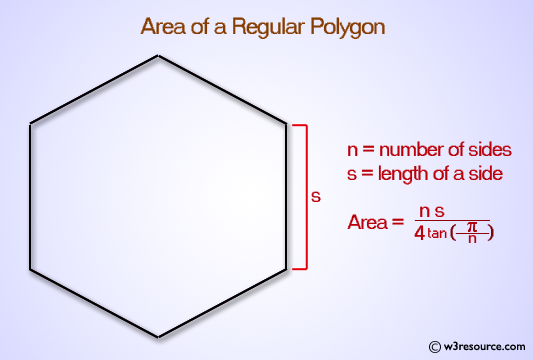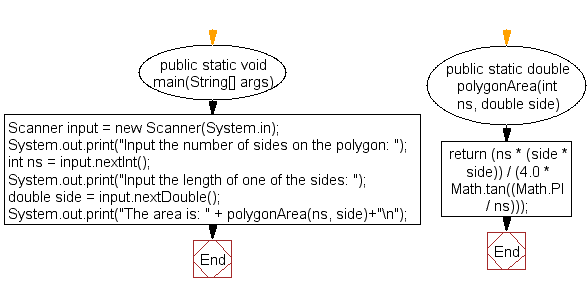﻿ Java exercises: Compute the area of a polygon - w3resource# Java Exercises: Compute the area of a polygon

## Java Basic: Exercise-35 with Solution

Write a Java program to compute the area of a polygon.

Area of a polygon = (n*s^2)/(4*tan(π/n))
where n is n-sided polygon and s is the length of a side.

Test Data:
Input the number of sides on the polygon: 7
Input the length of one of the sides: 6

Pictorial Presentation: Area of PolygonSample Solution:

Java Code:

``````import java.util.Scanner;

public class Exercise35 {

public static void main(String[] args) {

Scanner input = new Scanner(System.in);
System.out.print("Input the number of sides on the polygon: ");
int ns = input.nextInt();
System.out.print("Input the length of one of the sides: ");
double side = input.nextDouble();
System.out.print("The area is: " + polygonArea(ns, side)+"\n");
}
public static double polygonArea(int ns, double side) {
return (ns * (side * side)) / (4.0 * Math.tan((Math.PI / ns)));
}
}
```
```

Sample Output:

```Input the number of sides on the polygon: 7
Input the length of one of the sides: 6
The area is: 130.82084798405722
```

Flowchart:Java Code Editor:

What is the difficulty level of this exercise?

Test your Programming skills with w3resource's quiz.

﻿

## Java: Tips of the Day

countOccurrences

Counts the occurrences of a value in an array.

Use Arrays.stream().filter().count() to count total number of values that equals the specified value.

```public static long countOccurrences(int[] numbers, int value) {
return Arrays.stream(numbers)
.filter(number -> number == value)
.count();
}
```

Ref: https://bit.ly/3kCAgLb